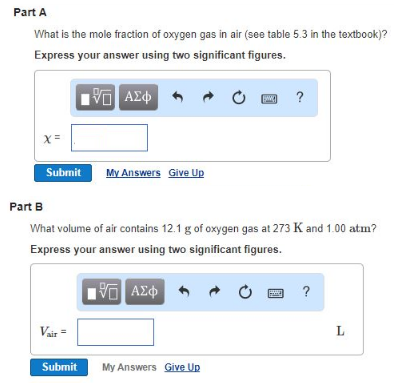# Problem: Part AWhat is the mole fraction of oxygen gas in air (see table 5.3 in the textbook)? Part BWhat volume of air contains 12.1 g of oxygen gas at 273 K and 1.00 atm? Express your answer using two significant figures.

###### FREE Expert Solution
100% (412 ratings)###### Problem Details

Part A

What is the mole fraction of oxygen gas in air (see table 5.3 in the textbook)?

Part B

What volume of air contains 12.1 g of oxygen gas at 273 K and 1.00 atm?